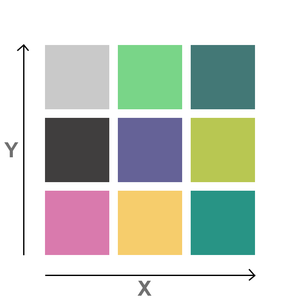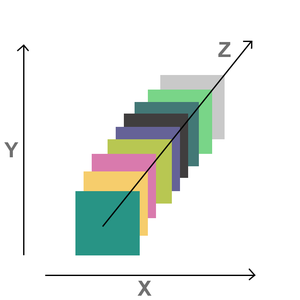Open in App
Not now

# How does z-index layer work ?

• Last Updated : 03 Jun, 2021

In this article, we will see how z-index works in CSS. As we all know that we take the help of dimension to look at every item, determines the size of an object completely. So z-index is used to make render the element along the z-axis. The default value of the z-index is zero.

### Example:

Let’s understand it from an example.

We have some cardboard that has the same size, but they are all different colors. So if we want to see them together, But will look something like this to us.2D

In this, we have used only two dimensions, that is, on the X dimension and the Y dimension, we can also see in it the Z dimension.3D

The whole concept of z-index is related to the 3D we can adjust the z-axis with the property called z-index.

The value of z can also be positive, negative, or zero.

## HTML

 ```<``html` `lang``=``"en"``>` `<``head``>``    ``<``link` `rel``=``"stylesheet"` `href``=``"style.css"``>``` `<``body``>``    ``<``div` `class``=``"d1"``>I'm Div One``    ``<``div` `class``=``"d2"``>I'm Div Two``    ``<``div` `class``=``"d3"``>I'm Div Three``    ``<``div` `class``=``"d4"``>I'm Div Four``` ``

## CSS

 `div {``    ``width``: ``140px``;``    ``height``: ``140px``;``    ``margin``: ``10px``;``    ``text-align``: ``center``;` `    ``/* Only display the last div */``    ``position``: ``absolute``;``}`` ` `/* If we want to adjust the z-axis */``/* use z-index */``.d``1` `{``    ``background-color``: ``#79D588``;``    ``top``: ``50px``;``    ``/* z-index: 10; */``    ``/* Use comment/uncomment upper``    ``line to see the difference */``}`` ` `.d``2` `{``    ``background-color``: ``#F6CD6C``;``    ``top``: ``70px``;``    ``/* z-index: 10; */``    ``/* Use comment/uncomment upper``    ``line to see the difference */``}`` ` `.d``3` `{``    ``background-color``: ``#289485``;``    ``top``: ``90px``;``    ``z-index``: ``10``;``    ``/* Use comment/uncomment upper``    ``line to see the difference */``}`` ` `.d``4` `{``    ``background-color``: ``#403E3E``;``    ``top``: ``110px``;``    ``/* z-index: 10; */``    ``/* Use comment/uncomment upper``    ``line to see the difference */``}`

Note: You can comment/uncomment the z-index properties of each div and then check the output one by one.

#### Output :output

My Personal Notes arrow_drop_up